# Intermediate Maths Solutions for 1A Functions Exercise 1(c)

Inter Maths 1A text book exercise 1(c) Functions solutions are given.

These solutions are very easy to understand. Study the text book lesson very well.

Then observe the example problems and solutions. Try them.

Observe the given solutions and try them in your own method.

You can also see

SSC Maths text book Solutions class 10

Inter Maths 1A Solutions textbook

Inter Maths 1B textbook solutions

Inter Maths 1IA text book solutions

Inter Maths IIB text book solutions

Functions

Exercise 1(a)

Exercise 1(b)

Exercise 1(c)

M

## Inter Maths Solutions for 1A Functions Exercise 1(c)

Exercise 1(c)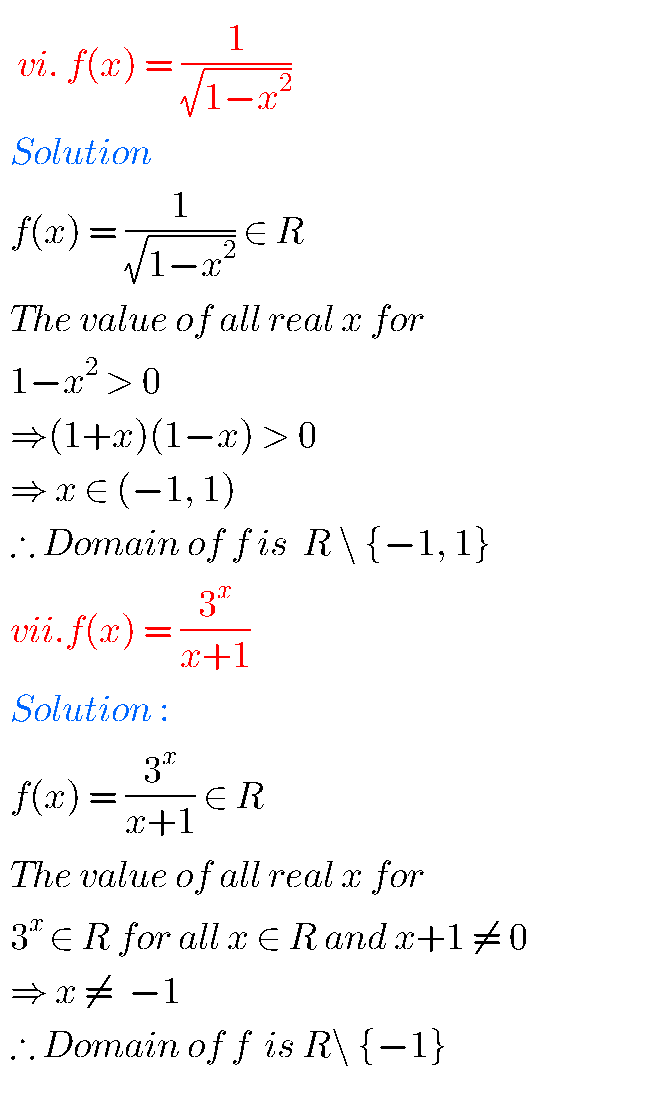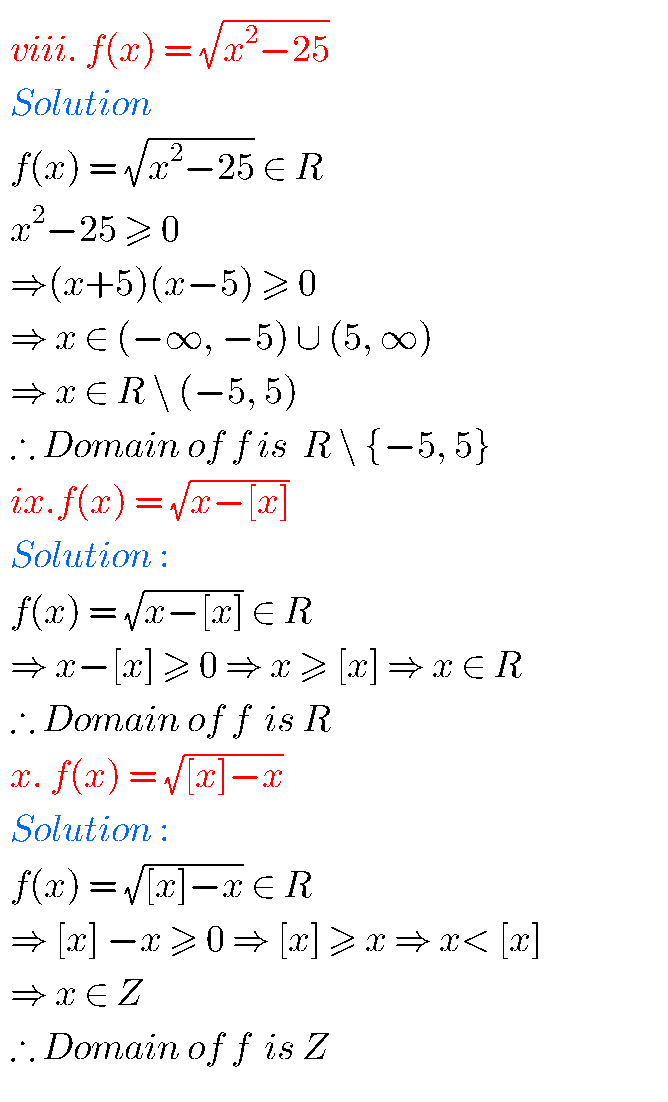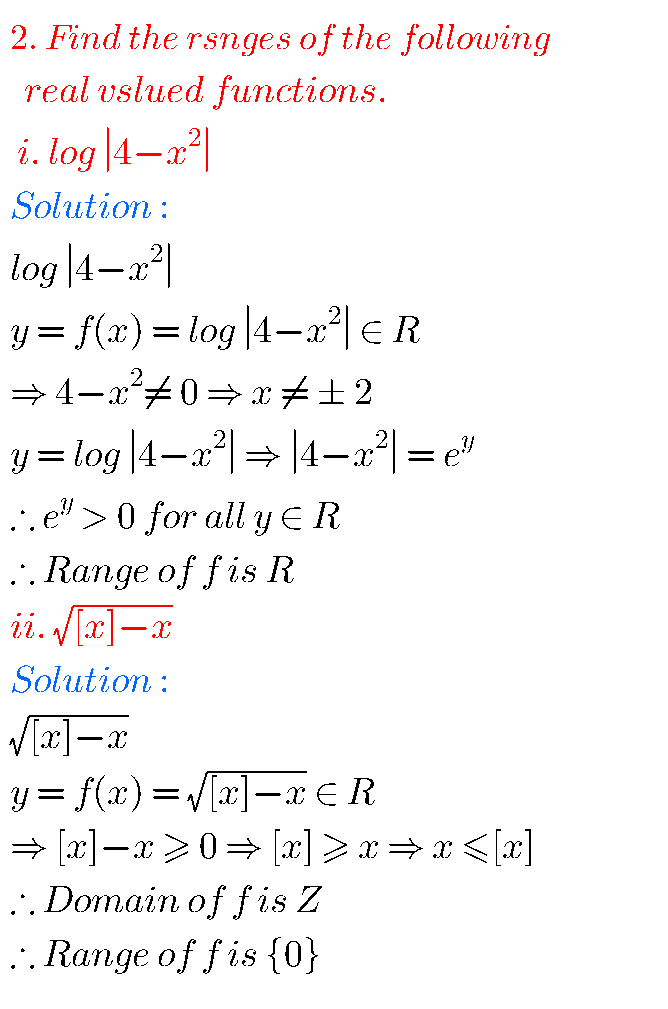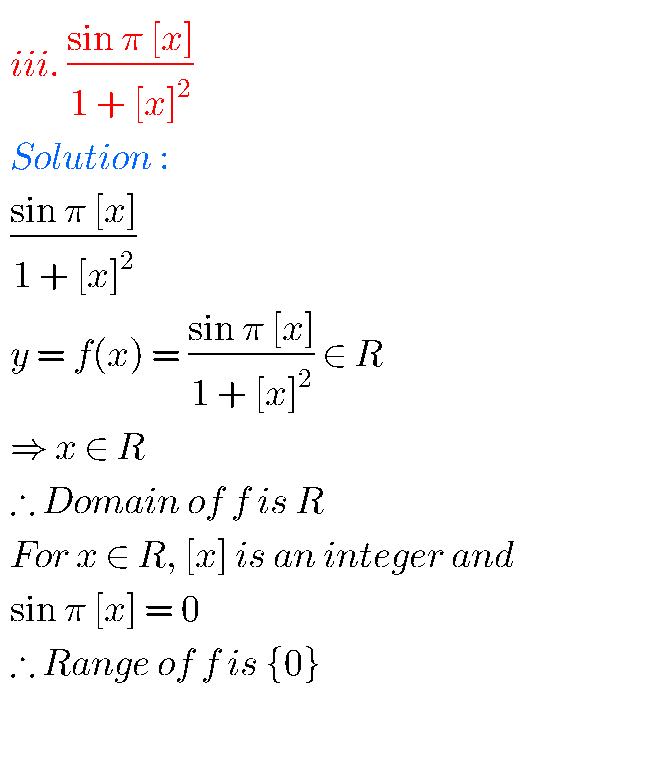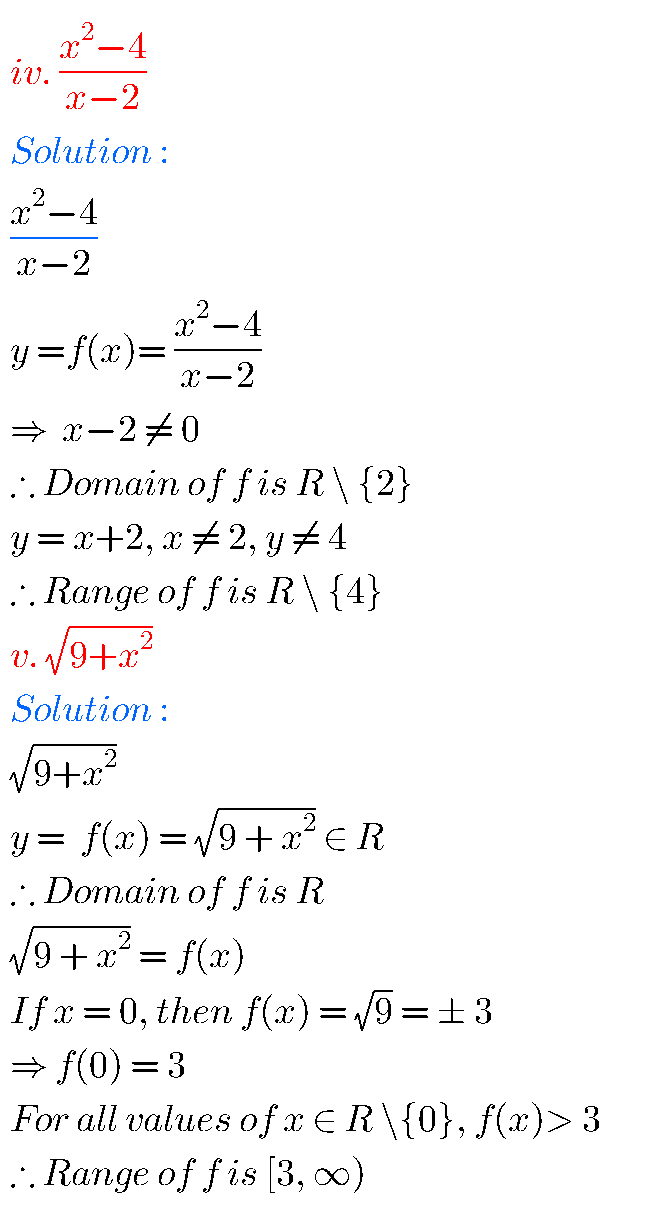## Maths 1A Solutions for exercise 1(c) Functions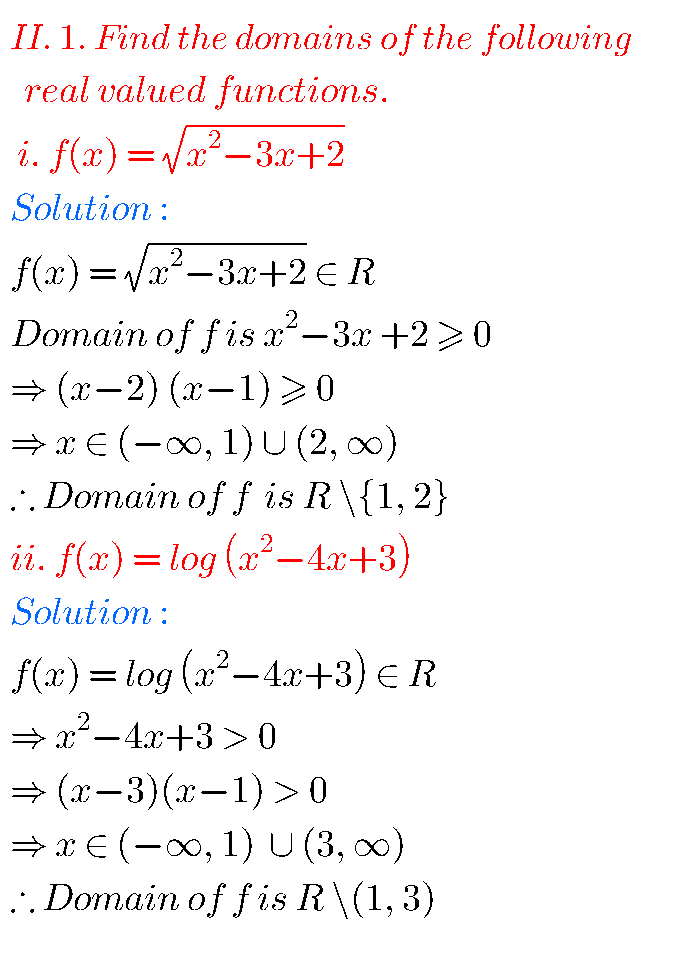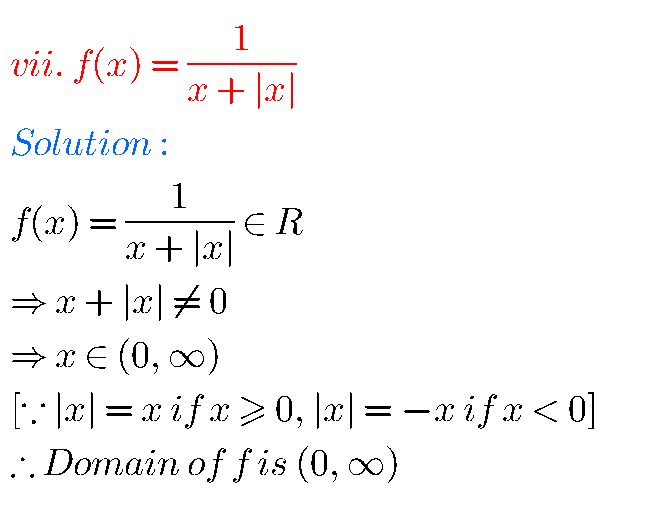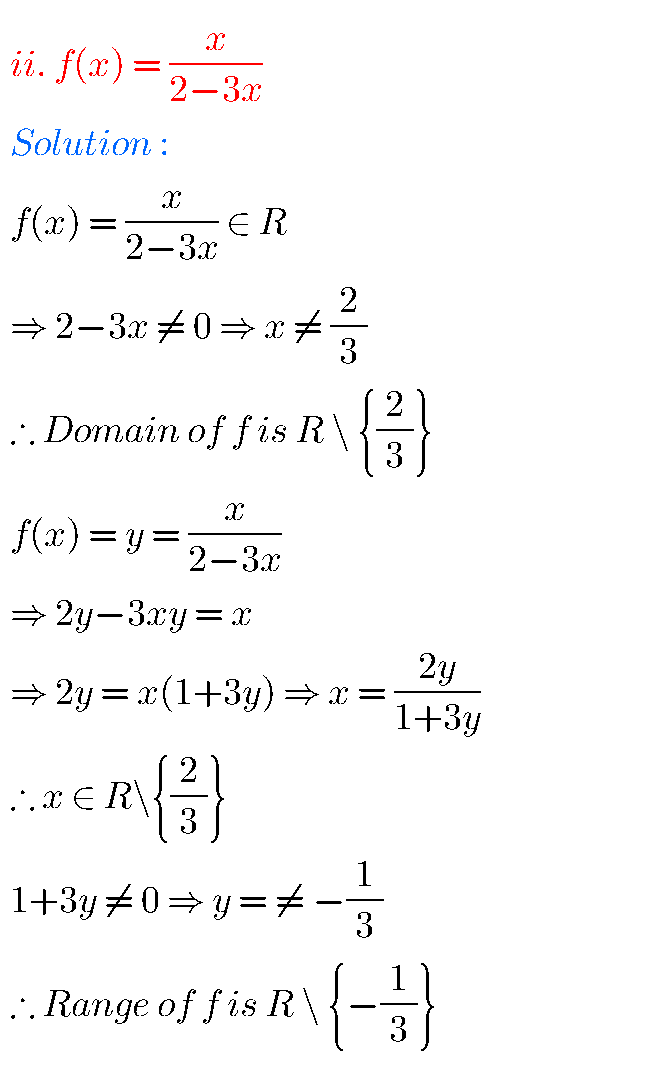Note : Observe the solutions and try them in your own method.

Some moreInter Maths solutions for Trigonometry

Nios Solutions for maths Senior secondary